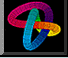# Math 210 - Spring 2018: Term Project suggestions

### Description of the term project

The term project part of the course consists of working with a group of four other students on a paper about a mathematical topic which you will present to the class.

Class presentations should be about 25 minutes long, so that we can have two presentations on a given day. The combination of your presentation and the paper you write with other members of your project team will count for 25 per cent grade.

Please have a look at the list of projects below and e-mail me an ordered list of your first three choices. I will then put people in touch with other class members interested in the same project. We will also set up a time so that everyone can meet with me to discuss their project. If you think of a mathematical project not on this list which you'd like to pursue, that would be fine. Please e-mail me about what you have in mind and we will meet to discuss this.

## An example of a presentation and write-up

In 2012 a group of students in math 210 decided to study the prevalence of the golden ratio in a particular painting by Mondrian. They modeled what the distribution of heights in comparison to widths in a painting which contained random" rectangles. They then compared this distribution with what the rectangles they saw in a painting of Mondrian. This gave some support to the idea that Mondrian did favor the golden ratio.

Here is their in-class presentation

Here is a write-up of their work.

## Possible term projects

1. ### The inverse B.S. problem

This problem is to determine to what extent the parameters in a 3 by 3 payoff matrix can be determined from knowing the optimal strategies in the two person zero sum game defined by this matrix. This is a natural question to ask when trying to understand from the behavior of the players what their motivations are. Suppose in the B.S. model discussed in homework #4, one allows the credibility constant c and the lying constant \ell to be different from each other. For which values of c and \ell are the values of c and \ell determined by the optimal strategies of the two players? You might first want to analyze the analogous problem for the 2 by 2 truth versus lying game.

2. ### The mathematics of choice

This project is about one model for how to make a choice from among a sequence of alternatives being considered. Imagine that you can interview a sequence of candidates for a job of some kind. After each interview you either have to decide to choose the candidate just interviewed or move on the next, and if you move on then you can't return to choose an earlier candidate. What is the best strategy to use if you want to maximize the chances of ending up with the best candidate? What if you want to maximize the expected rank of the candidate you choose?

3. ### Mathematical sociology

This project has to do with trying to understand social behavior mathematically. Here is one example. Suppose that every pair of people in a group of people must declare whether they are enemies or friend. Suppose further that the friend of one's friend must be a friend, and the enemy of one's enemy must be one's friend. Finally suppose that no three people are all enemies of one another; in other words, an alliance between two of the three always develops. Then one can show the entire population can be divided into two groups, with members of each group being friends with members of the same group and enemies of all members of the other group.

4. ### The theory of information

This project is about Shannon's entropy function, which measures the expected amount of information in a message or inherent in a network. Conditional Shannon entropy measures how much more information is expected from a new message, given the data of messages already received. It would be interesting to apply these concepts to the media.

5. ### How not to be wrong

There are many potential final project topics in the new book How not to be wrong" by Jordan Elleberg. Some examples are: How some college students beat the Massachussetts state lottery and how Facebook could detect if your neighbor is a terrorist. If you find some chapters in this book interesting, send me an e-mail and we can talk about how to build a project around them. Since the book was written for a popular audience, we would need to augment this with some further mathematical details using what we have been doing in the course.

6. ### The evolution of cooperation

The game theory models which we have discussed in class generally considered situations in which two or more people would interact exactly once. If the same people interact many times, different strategies may become optimal. This project has to do with understanding the research on this topic and its relevance to social and biological behavior. Here is an untidy Wikipedia article about "The evolution of cooperation" by Robert Axelrod.
Last updated: 3/12/18
Send e-mail comments to: ted@math.upenn.edu